Calculus of Single & Multiple Variables -Assignment

# Calculus of Single & Multiple Variables -Assignment - Notes | Study Mathematical Models - Physics

 1 Crore+ students have signed up on EduRev. Have you?

Q.1. If f (x) be a twice differentiable function such that f"(x) = -f (x) and f'(x) = g (x ). If h'(x) = [f(x)]2+[g(x)]2 ’h (1) = 8 and h (0) = 2 then find the value of h (2) .
Ans. h'( X ) = [f ( x )]2+[ g ( x )]2
h'' ( x ) = 2 f (x ) f' ( x ) + 2 g ( x ) g ' (x )
h'' ( x ) = 2 f(x) g (x ) + 2g ( x ) f'' (x )
h'' ( x ) = 2 f(x) g (x ) + 2g ( x ) (-f (x )) = 0
Thus, h'(x) = c, a constant for all x.
⇒ h (x) = cx + c1
It is given that h (l) = 8 and h (0) = 2, therefore c1 = 2 and c2 = 6
∴ h (x ) = 6 x + 2 ⇒ h (2) = 6 x 2 + 2 = 14

Q.2. If y = ax then find the value of dy/dx as a function of x and y ( a is a constant).
Ans. We can write y = axy
Taking Logrithm of both sides, we obtain ln y = xy ln a.
⇒ ln (ln y) = y ln x + ln (ln a)
Differentiating with respect to x gives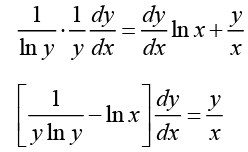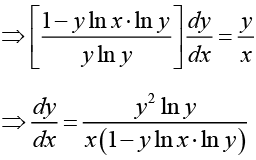Q.3. Find the co-ordinates of the point P on the curve y2 = 2x3 such that the tangent at P is perpendicular to the line 4 x - 3 y + 2 = 0.
Ans.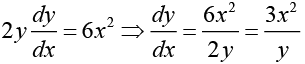Let, P(x0,y0) be the point at which the tangent is perpendicular to the line 4x - 3y + 2 = 0.
Thus slope at P is equal to m =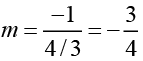Thus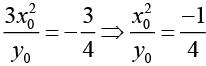(i)
From the equation of curve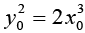(ii)
From equation (i) and (ii)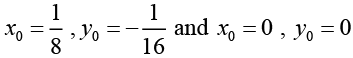Thus there are two points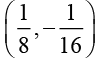and (0,0) at which the tangent line is perpendicular
to the line 4x - 3y + 2 = 0.

Q.4. If the tangent to the curve xy + ax + by = 0 at (1,1) is inclined at an angle tan-1 2 with x axis then find the values of a and b .
Ans. The point (1,1) lies on the curve xy + ax + by = 0.
Hence, a+b = -1    (i)
Differentiating xy + ax + by = 0 with respect to x we get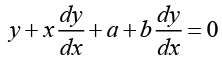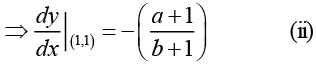Since the tangent at (1,1) makes an angle of tan-1 2 with the x - axis hence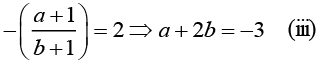Solving equations (i) and (ii) we obtain
a = 1 and b = -2

Q.5. If f (x) = a log |x| + bx2 + x has its extreme values (local maximum or minimum value) at x = -1 and x = 2 , then find the values of a and b .
Ans. The logarithmic function is defined for all x > 0. Hence the domain of f (x) is (0, ∞) . Using this fact we can write
f (x) = a log x + bx2 + x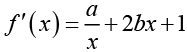We see that the derivative is defined at all points of the domain of the function. Hence the function can attain extreme values only at points where f'(x) = 0. Since f (x) attains its extreme values at x = -1,2.
∴ f'(-1) = 0 and f'(2) = 0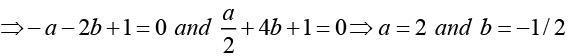Q.6. If y = sin-1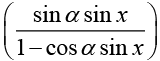where α is a constant then find the value of y'(0) .

Ans.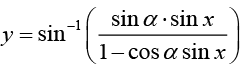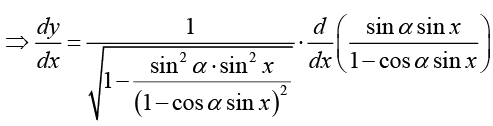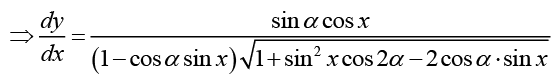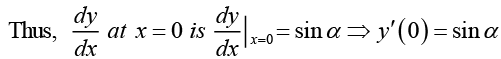Q.7. Find the equation of the tangent to the curve x = t cos t and y = t sin t at the origin of xy plane.
Ans.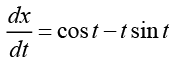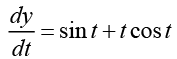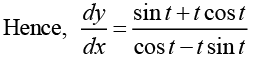At the origin x = 0, y = 0
⇒ t cos t = 0 and t sin t = 0
t = 0 and cos t = 0 and t = 0 and sin t = 0
Since there is no value of t for which cos t and sin t are simultaneously 0 , hence t = 0.Thus the slope of the tangent at (0,0) is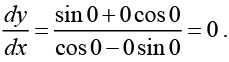Thus the equation of the tangent at the origin is y = 0.

Q.8. A curve with equation of the form y = ax4 + bx3 + c + cx + d has slope 0 at the point (0,1) and also touches the x -axis at the point (-1,0) then find the values of x for which the curve has negative slope.
Ans: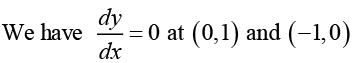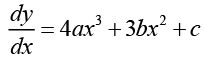Hence, 4a • 0+3b • 0+c = 0 ⇒ c = 0
And, 4a (-1) + 3b = 0    (i)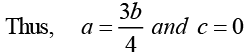Also, the curve passes through (0,1) and (-1,0), hence
d = 1 and 0 = a - b - c + d
⇒ a - b - c + 1 = 0    (ii)
From (i) and (ii), we get
a = 3,b = 4,c = 0 d = 1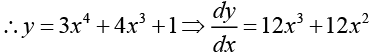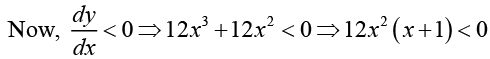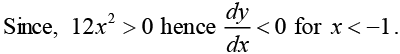Q.9. Find the point at which the local maximum or local minimum value of the function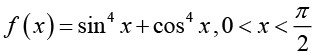occurs. Also find the local minimum and for maximum values of the function.
Ans. We have y = f (x) = sinx + cos4 x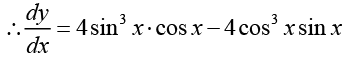= -4 cos x sin x (cos2 x - sin2 x)
= -2 sin 2x cos 2x = - sin 4x
Over the given interval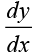is defined for all x , hence for local maximum or minimum value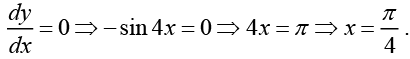Where we have used the fact that.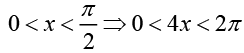Thus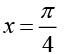is the only point where local maximum or minimum value of the function occurs. Here we can use either first derivative test or the second derivative test to check for local maxima or minimum. Using the second derivative test, we obtain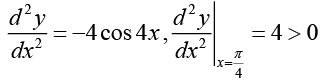Thus at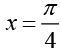a local minimum occurs. The local minimum value is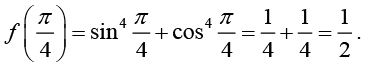Note: Students should check using the first derivative test that a local minimum indeed occurs at x =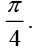Q.10. If A > 0,B > 0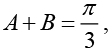then find the maximum value of tan A + tan B.
Ans. We have A + B =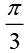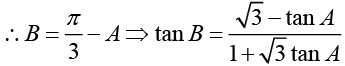Let, z = tan A+tan B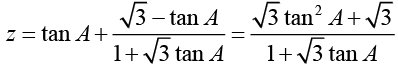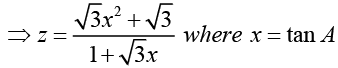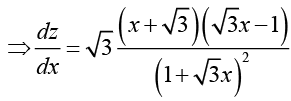F or maximum value of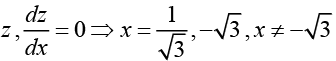because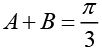which implies that x = tan A > 0. It can be earily checked that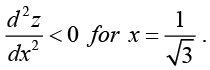Hence, z is maximum for x =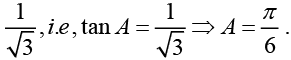For this value of x, z =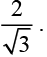The document Calculus of Single & Multiple Variables -Assignment - Notes | Study Mathematical Models - Physics is a part of the Physics Course Mathematical Models.
All you need of Physics at this link: Physics

## Mathematical Models

18 docs|24 tests
 Use Code STAYHOME200 and get INR 200 additional OFF

## Mathematical Models

18 docs|24 tests

Track your progress, build streaks, highlight & save important lessons and more!

,

,

,

,

,

,

,

,

,

,

,

,

,

,

,

,

,

,

,

,

,

;